# NCERT Solutions for Class 6 Maths Chapter 5 Understanding Elementary Shapes

NCERT solutions for class 6 maths chapter 5 Understanding Elementary Shapes- All the shapes that you see around yourself are formed using lines and curves. You can see edges, planes, corners, closed curves and open curves in our surroundings. You can organize them into line, line segments, angles, circles triangles, and polygons. Solutions of NCERT for class 6 maths chapter 5 Understanding Elementary Shapes are covering problems related to all the above-mentioned figures. You know that all these have different measures and sizes. This chapter deals with the development of tools to measure shapes like triangles, polygons, etc and their sizes. It is one of the most important chapters of this class as well as of the geometry part. So, to have a command on the topic, you, must pay attention to this chapter while studying. Important subtopics covered under this chapter are- measuring line segments, angles – ‘Acute’, ‘Obtuse’ and ‘Reflex’, angles – ‘Right’ and ‘Straight’, measuring angles, perpendicular lines, classification of triangles. CBSE NCERT solutions for class 6 maths chapter 5 Understanding Elementary Shapes is covering the solution from each of the subtopics. In this chapter, there are 45 questions in 9 exercises. Solutions to all these 45 problems are covered comprehensively in solutions of NCERT for class 6 maths chapter 5 Understanding Elementary Shapes. Along with all these, if any time, you are in need of NCERT solutions for other classes and subjects then you can get it through the given link.

Go to the respective exercise on clicking the link below

Exercise 5.1

Exercise 5.2

Exercise 5.3

Exercise 5.4

Exercise 5.5

Exercise 5.6

Exercise 5.7

Exercise 5.8

Exercise 5.9

NCERT solutions for class 6 maths chapter 5 Understanding Elementary Shapes Exercise: 5.1

The disadvantage in comparing line segments by mere observation is that our estimation may be inaccurate and therefore a divider must be used.

While measuring the length of a line segment using error might creep in due to the thickness and translucency of the ruler and because of angular viewing. We can get rid of these errors using a divider.

[Note : If A,B,C are any three points on a line such that AC + CB = AB, then we can be sure that C lies between A and B.]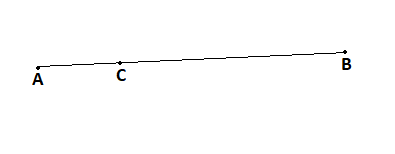Yes

AB = 5 cm

BC = 3 cm

AC = 8 cm

Therefore AB + BC = AC

Therefore point B lies between points A and C.AD = 4 - 1 = 3

DG = 7 - 4 = 3

Therefore D is the midpoint of AG.

To Prove

B is the mid point of AC

C is the mid point of BD

From (i) and (ii) we can conclude

Hence proved.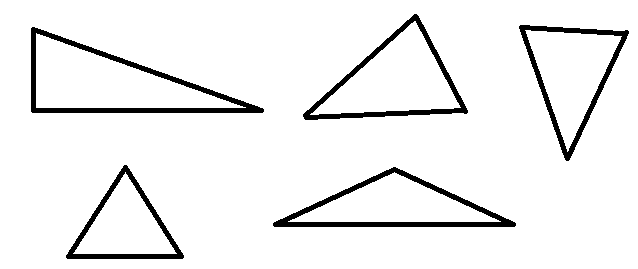After measuring their sides we have found that the sum of lengths of any two sides of a triangle is always greater than the third side.

## NCERT solutions for Topic: Angles

### Q1 What is the angle name for half a revolution?

Half a revolution =

The angle name for half a revolution is "Straight Angle".

## Q2 What is the angle name for one-fourth revolution?

One-fourth revolution =

The angle name for one-fourth revolution is "Right Angle"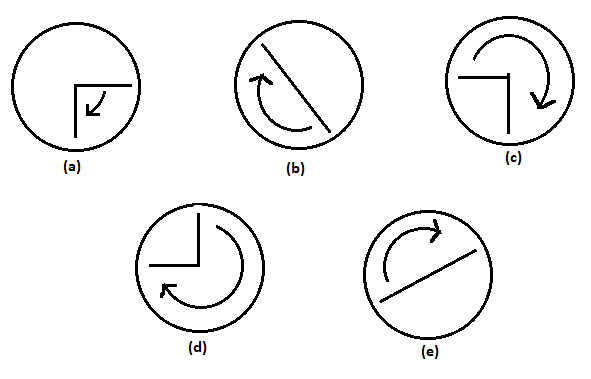(a) One fourth revolution: From

(b) Half revolution: From

(c) Three fourth revolution: From

(d) Three fourth revolution: From

(e) Half fourth revolution: From

## NCERT solutions for class 6 maths chapter 5 Understanding Elementary Shapes Exercise: 5.2

(a) 3 to 9                       (b) 4 to 7                  (c) 7 to 10

(d) 12 to 9                     (e) 1 to 10                (f) 6 to 3

(a) Half.

(b) One fourth.

(c) One fourth.

(d) Three fourth.

(e) Three fourth.

(f) Three fourth.

(a) starts at 12 and makes     of a revolution, clockwise?

(b) starts at 2 and makes     of a revolution, clockwise?

(c) starts at 5 and makes    of a revolution, clockwise?

(d) starts at 5 and makes     of a revolution, clockwise?

(a) The hand of a clock will stop at 6 after starting at 12 and making    of a revolution, clockwise.

(b) The hand of a clock will stop at 8 after starting at 2 and making    of a revolution, clockwise.

(c) The hand of a clock will stop at 8 after starting at 5 and making    of a revolution, clockwise.

(d) The hand of a clock will stop at 2 after starting at 5 and making    of a revolution, clockwise.

(a) east and make    of a revolution clockwise?

(b) east and make     of a revolution clockwise?

(c) west and make    of a revolution anti-clockwise?

(d) south and make one full revolution?

(Should we specify clockwise or anti-clockwise for this last question? Why not?)

(a) West.

(b) West.

(c) North.

(d) South.

No need to specify clockwise or anti-clockwise for the last question as after one complete revolution we would be facing in the same direction.

(a) east and turn clockwise to face north?

(b) south and turn clockwise to face east?

(c) west and turn clockwise to face east?

(a) If we are standing facing east and turn clockwise to face north we have turned through  of a revolution.

(b)  If we are standing facing south and turn clockwise to face east we have turned through   of a revolution.

(c) If we are standing facing west and turn clockwise to face east we have turned through half of a revolution.

(a) 3 to 6                                 (b) 2 to 8                          (c) 5 to 11

(d) 10 to 1                               (e) 12 to 9                        (f) 12 to 6

Number of right angles turned through by the hour hand of a clock when it goes from

(a) 3 to 6,   (b) 2 to 8,   (c) 5 to 11,  (d) 10 to 1,   (e) 12 to 9,  (f) 12 to 6  are

(a) 1.

(b) 2.

(c) 2.

(d) 1.

(e) 3.

(f) 2.

(a) south and turn clockwise to west?

(b) north and turn anti-clockwise to east?

(c) west and turn to west?

(d) south and turn to north?

The number of right angles we can make from the given conditions are-

(a) 1.

(b) 3.

(c) 4.

(d) 2.

(a) from 6 and turns through 1 right angle?

(b) from 8 and turns through 2 right angles?

(c) from 10 and turns through 3 right angles?

(d) from 7 and turns through 2 straight angles?

(a) Starting from 6 and turns through 1 right angle the hour hand stops at 9.

(b) Starting from 8 and turns through 2 right angle sthe hour hand stops at 2.

(c) Starting from 10 and turns through 3 right angle the hour hand stops at 7.

(d) Starting from 7 and turns through 2 straight angle the hour hand stops at 7.

## NCERT solutions for Topic: Angles

### Q1 The hour hand of a clock moves from 12 to 5. Is the revolution of the hour hand more than 1 right angle?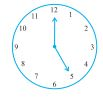Yes, the revolution of the hour hand is more than 1 right angle.

For each hour, angle made =

Therefore, when the hour hand moves from 12 to 5, the angle made =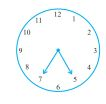No, the angle is not more than than 1 right angle.

For each hour, angle made =

Therefore, when the hour hand moves from 5 to 7, the angle made =

## NCERT solutions for class 6 maths chapter 5 Understanding Elementary Shapes Exercise: 5.3

 (i) Straight angle (a) Less than one-fourth of a revolution (ii) Right angle (b) More than half a revolution (iii) Acute angle (c) Half of a revolution (iv) Obtuse angle (d) One-fourth of a revolution (v) Reflex angle (e) Between    and    of a revolution (f) One complete revolution

 (i) Straight angle (c) Half of a revolution (ii) Right angle (d) One-fourth of a revolution (iii) Acute angle (a) Less than one-fourth of a revolution (iv) Obtuse angle (e) Between    and    of a revolution (v) Reflex angle (b) More than half a revolution(a) Acute.

(b) Obtuse.

(c) Right.

(d) Reflex.

(e) Straight.

(f) Acute, acute.

## NCERT solutions for class 6 maths chapter 5 Understanding Elementary Shapes Exercise: 5.4

(i) 90o

(ii) 180o

(a) some acute angles.

(b) some obtuse angles.

(give at least two examples of each).

(a) 30o, 45o and 60o

(b) 120o, 135o and 150o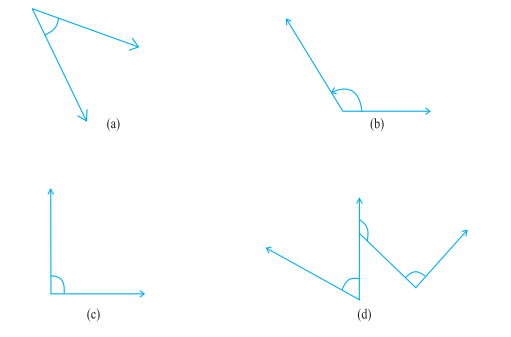(a) 45o

(b) 125o

(c) 90o

(d) 60o, 90o and 125oMeasure of Angle A =

Measure of Angle B =

Measure of Angle A = 40o

Measure of Angle B = 60oBy estimation followed by confirmation by measurement we know that the second angle is greater.

(a) An angle whose measure is less than that of a right angle is______.

(b) An angle whose measure is greater than that of a right angle is ______.

(c) An angle whose measure is the sum of the measures of two right angles is _____.

(d) When the sum of the measures of two angles is that of a right angle, then each one of them is ______.

(e) When the sum of the measures of two angles is that of a straight angle and if one of them is acute then the other should be _______.

(a) An angle whose measure is less than that of a right angle is acute.

(b) An angle whose measure is greater than that of a right angle is obtuse.

(c) An angle whose measure is the sum of the measures of two right angles is straight.

(d) When the sum of the measures of two angles is that of a right angle, then each one of them is acute.

(e) When the sum of the measures of two angles is that of a straight angle and if one of them is acute then the other should be  obtuse.(a) Measure of the given along = 40o

(b) Measure of the given along = 130o

(c) Measure of the given along = 65o

(d) Measure of the given along = 135oThe angle measure between the hands of the clock in each figure is

(a) 90o

(b) 30o

(c) 180oANGLE MEASURE TYPE

 ANGLE MEASURE TYPE 40o Acute Angle 125o Obtuse Angle 85o Acute Angle 95o Obtuse Angle 1400 Obtuse Angle 1800 Straight Angle

## NCERT solutions for class 6 maths chapter 5 Understanding Elementary Shapes Exercise: 5.5

(a) The adjacent edges of a table top.

(b) The lines of a railway track.

(c) The line segments forming the letter ‘L’.

(d) The letter V.

(a) The adjacent edges of a table top are models for perpendicular lines.

(b) The lines of a railway track are not models for perpendicular lines as they are parallel to each other.

(c) The line segments forming the letter ‘L’ are models for perpendicular lines.

(d) The line segments forming the letter ‘V’ are models for perpendicular lines.

PQ and XY intersect at A

Therefore

The angles of the two set squares are

(i) 90o, 60o and 30o

(ii) 90o, 45o, and 45o

Yes they have the common angle measure 90o

(a) Is CE = EG?

(b) Does PE bisect CG?

(c) Identify any two line segments for which PE is the perpendicular bisector.

(d) Are these true?

(i) AC > FG

(ii) CD = GH

(iii) BC < EH.(a) CE = 5 - 3 = 2 units

EG = 7 - 5 = 2 units

Therefore CE = EG.

(b) CE = EG therefore PE bisects CG.

(c) PE is the perpendicular bisector for line segments DF and BH

(d) (i) AC = 3 - 1 = 2 units

FG = 7 - 6 = 1 unit

Therefore AC > FG

True

(ii) CD = 4 - 3 = 1 unit

GH = 8 - 7 = 1 unit

Therefore CD = GH

True

(iii) BC = 3 - 2 = 1 unit

EH = 8 - 5 = 3 units

Therefore BC < EH

True.

## NCERT solutions for Topic: Classification of Triangles

(a) a scalene acute angled triangle.

(b) an obtuse angled isosceles triangle.

(a) a scalene acute angled triangle. :

Scalene : All side of different length

Acute angled : All angles less than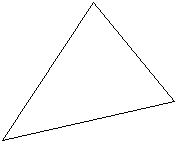(b) an obtuse angled isosceles triangle

Isosceles traingle: Only two sides are of equal length

Obtuse angled : At least one angle greater than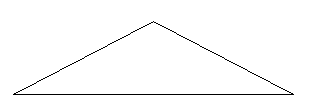## NCERT solutions for class 6 maths chapter 5 Understanding Elementary Shapes Exercise: 5.6

(a) Triangle with lengths of sides 7 cm, 8 cm and 9 cm.

(b)  with AB = 8.7 cm, AC = 7 cm and BC = 6 cm.

(c)  such that PQ = QR = PR = 5 cm.

(d)  with

(e)  with  and XY = YZ.

(f)  with ,  and

(a) Scalene Triangle.

(b) Scalene Triangle.

(c) Equilateral Triangle.

(d) Right-angled Triangle.

(e) Right-angled isosceles Triangle.

(f) Acute angled Triangle.

 Measure of triangles Types of triangle (i) 3 sides of equal length (a) Scalene (ii) 2 sides of equal length (b) Isosceles right-angled (iii) All sides of different length (c) Obtuse angled (iv) 3 acute angles (d) Right-angled (v) 1 right angle (e) Equilateral (vi) 1 obtuse angle (f) Acute angled (vii) 1 right angle with two sides of equal length (g) Isosceles

 Measure of triangles Types of triangle (i) 3 sides of equal length (e)Equilateral (ii) 2 sides of equal length (g) Isoscles (iii) All sides of different length (a) Scalene (iv) 3 acute angles (f) Acute angled (v) 1 right angle (d)Right angled (vi) 1 obtuse angle (c) Obtuse angled (vii) 1 right angle with two sides of equal length (b) Isoscles right angled(a)(i) Acute angled triangle.

(ii) Isosceles triangle.

(b)(i) Right-angled triangle.

(ii) Scalane triangle.

(c)(i) Obtuse angled triangle.

(ii) Isosceles triangle.

(d)(i) Right-angled triangle.

(ii) Isosceles triangle.

(e)(i) Acute angled triangle.

(ii) Equilateral triangle.

(f)(i) Obtuse angled triangle.

(ii) Scalene triangle.

## NCERT solutions for Topic: Quadrilaterals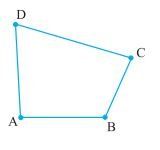The sides of the quadrilateral are AB, BC, CD, DA

The angles are given by

(a) all the four angles are acute.

(b) one of the angles is obtuse.

(c) one of the angles is right angled.

(d) two of the angles are obtuse.

(e) two of the angles are right angled.

(f) the diagonals are perpendicular to one another

(a) all the four angles are acute.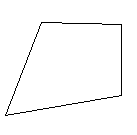(b) one of the angles is obtuse.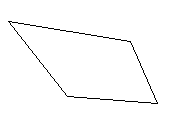(c) one of the angles is right angled.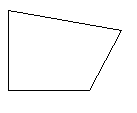(d) two of the angles are obtuse.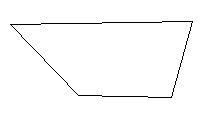(e) two of the angles are right angled.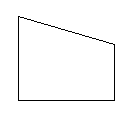(f) the diagonals are perpendicular to one another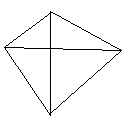A square can be thought of as a special rectangle as it is a rectangle only but with all sides equal.

A rectangle can be thought of as a special parallelogram as it s a parallelogram only but with all angles equal to ninety degrees.

A square can be thought of as a special rhombus because like a rhombus it has all sides equal but all its angles are also equal.

Squares, rectangles, parallelograms are all quadrilaterals as they all have four sides.

Square is also a parallelogram as its opposite sides are parallel.

Square is the only quadrilateral with sides equal in length and angles equal in measure, therefore, a square is the regular quadrilateral.

## NCERT solutions for class 6 maths chapter 5 Understanding Elementary Shapes Exercise: 5.8(a) The given figure is not a polygon as it is not a closed figure.

(b) The given figure is  a polygon.

(c) The given figure is not a polygon as a polygon is enclosed only by line segments.

(d) The given figure is not a polygon as a polygon is enclosed only by line segments.Make two more examples of each of these.(b) Triangle(c) Pentagon(d) OctagonWe have drawn the regular Hexagon ABCDEF and by joining the vertices B, D and F we have formed the Equilateral Triangle BDF.We have made the regular octagon ABCDEFGH and by joining vertices H, C, D and G we have formed the rectangle HCDGWe have drawn the pentagon ABCDE and by joining its vertices he has drawn the diagonals AC, CE, EB, BD and DA.

## NCERT solutions of class 6 maths chapter 5 Understanding Elementary Shapes Topic: Three Dimensional Shapes

It has ______ faces.

Each face has ______ edges.

Each face has ______ vertices.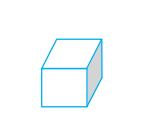It has  faces.        (Three pairs of parallel square faces)

Each face has  edges.

Each face has  vertices

Faces : _______

Edges : _______

Corners : _______The number of

Faces =           (All triangular faces)

Edges =

Corners =

Faces : _______

Edges : _______

Corners : _______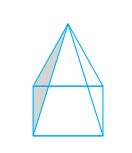In a square pyramid, the number of

Faces =           (Four triangular faces and one square face)

Edges =           (Four edges of the square base and other four joining at the top)

Corners =

Faces : _______

Edges : _______

Corners : _______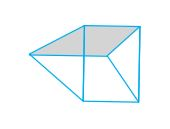Faces =   (Two triangular faces and three square faces)

Edges = 9

Corners =

## NCERT solutions for class 6 maths chapter 5 Understanding Elementary Shapes Exercise: 5.9

(a) Cone                                                          (i)(b) Sphere                                                      (ii)(c) Cylinder                                                     (iii)(d) Cuboid                                                       (iv)(e) Pyramid                                                      (v)(a) Cone                                                  (ii)(b) Sphere                                               (iv)(c) Cylinder                                              (v)(d) Cuboid                                             (iii)(e) Pyramid                                            (i)the shape of the following things are

(b) A brick- Cuboid

(c) A matchbox-Cuboid

## NCERT solutions for class 6 mathematics chapter-wise

 Chapters No. Chapters Name Chapter - 1 NCERT solutions for class 6 maths chapter 1 Knowing Our Numbers Chapter - 2 Solutions of NCERT for class 6 maths chapter 2 Whole Numbers Chapter - 3 CBSE NCERT solutions for class 6 maths chapter 3 Playing with Numbers Chapter - 4 NCERT solutions for class 6 maths chapter 4 Basic Geometrical Ideas Chapter - 5 Solutions of NCERT for class 6 maths chapter 5 Understanding Elementary Shapes Chapter - 6 CBSE NCERT solutions for class 6 maths chapter 6 Integers Chapter - 7 NCERT solutions for class 6 maths chapter 7 Fractions Chapter - 8 Solutions of NCERT for class 6 maths chapter 8 Decimals Chapter - 9 CBSE NCERT solutions for class 6 maths chapter 9 Data Handling Chapter -10 NCERT solutions for class 6 maths chapter 10 Mensuration Chapter -11 Solutions of NCERT for class 6 maths chapter 11 Algebra Chapter -12 CBSE NCERT solutions for class 6 maths chapter 12 Ratio and Proportion Chapter -13 NCERT solutions for class 6 maths chapter 13 Symmetry Chapter -14 Solutions of NCERT for class 6 maths chapter 14 Practical Geometry

## NCERT solutions for class 6 subject wise

 NCERT Solutions for class 6 maths Solutions of NCERT for class 6 science

## How to use NCERT solutions for class 6 maths chapter 5 Understanding Elementary Shapes?

• You must have covered the previous chapter Basic Geometrical Ideas.
• Read the conceptual text given in the NCERT textbook.
• Learn the application of all these concepts in the problems.
• When you are done with all the above three points, you can practice it.
• While practicing you can utilize solutions of NCERT for class 6 maths chapter 5 Understanding Elementary Shapes.

Keep learning and working hard!